Question

Carrie has 40 more nickels than Joan has dimes. They both have the same amount of money. How many coins does each girl have? What is their total amount of money?

1.Carrie and Joan have 80 nickels and 40 dimes, respectively, and each one has an amount of money of 4 US dollars.

Step-by-step explanation:

Let be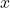and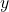the amounts of nickels and dimes that Carrie and Joan have, as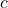the total amount of money that both Carrie and Joan have. A nickel is a five cent coin and a dime is a ten cent coin.

The following equations are constructed after a careful reading on statement:

Carrie’s amount of money: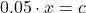(Eq. 1)

Joan’s amount of money:(Eq. 2)

Relation between amounts of coins: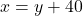(Eq. 3)

First we eliminateby equalizing (Eq. 1) and (Eq. 2):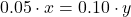Then, we reduce the resulting formula by (Eq. 3):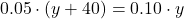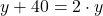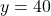And rest of variable are now determined: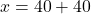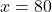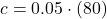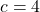Carrie and Joan have 80 nickels and 40 dimes, respectively, and each one has an amount of money of 4 US dollars.